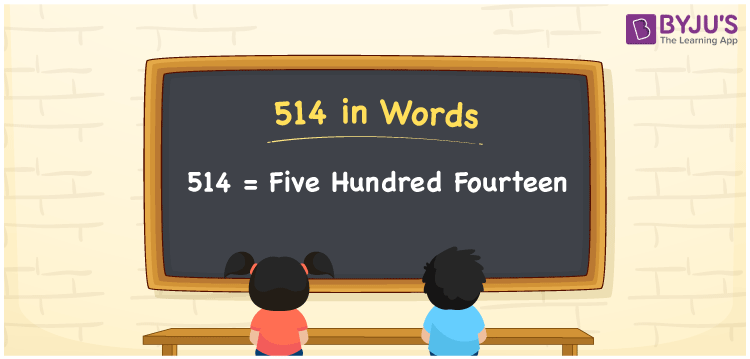# 514 in Words

514 in Words is written as Five hundred fourteen. We can say that Five hundred fourteen is the number name for 514. We generally use the number names to express or communicate those numbers to others. Suppose you are asked to read the amount Rs. 514, you may read it as “Five hundred fourteen rupees”. However, you can learn how to derive the word form of 514 here in this article.

 514 in words Five hundred fourteen Five hundred fourteen in Numbers 514

## 514 in English Words

Generally, we write numbers in words using the English alphabet. So, we express 514 in English words as “Five hundred fourteen”.## How to Write 514 in Words?

As we can see, 514 is a three-digit number so, let’s create a place value chart with three columns as shown below.

 Hundreds Tens Ones 5 1 4

Here, ones = 4, tens = 1, hundreds = 5

By expanding the above digits, we get;

5 × Hundred + 1 × Ten + 4 × One

= 5 × 100 + 1 × 10 + 4 × 1

= 500 + 10 + 4

= Five hundred + Ten + Four

= Five hundred fourteen {since ten + four = fourteen)

Therefore, 514 in words = Five hundred fourteen

We know that 514 is a natural number that precedes 515 and succeeds 513.

514 in words – Five hundred fourteen

Is 514 an even number? – Yes

Is 514 an odd number? – No

Is 514 a prime number? – No

Is 514 a composite number? – Yes

Is 514 a perfect square number? – No

Is 514 a perfect cube number? – No

## Frequently Asked Questions on 514 in Words

Q1

### How do you write 514 in words?

We can write the number 514 in words as Five hundred fourteen.
Q2

### Write 514.50 in words.

The number 514.50 can be written in words as “Five hundred fourteen point five zero”.
Q3

### How to write Rs. 514 in words on a cheque?

On a cheque, we generally write Rs. 514 in words as “Five hundred fourteen rupees only”.